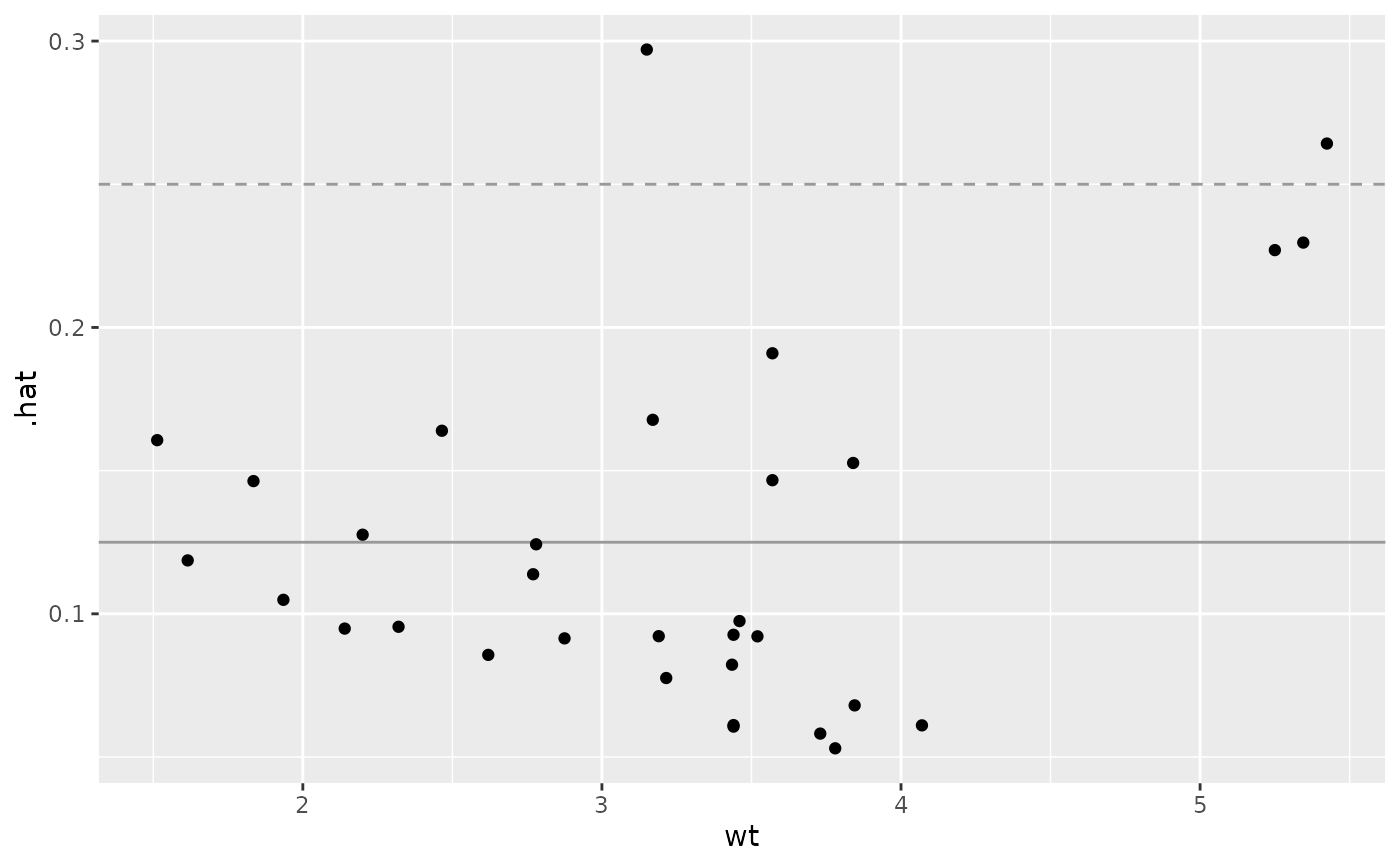A function to display stats::influence's hat information against a given explanatory variable.

ggally_nostic_hat(
data,
mapping,
...,
linePosition = 2 * sum(eval_data_col(data, mapping$y))/nrow(data), lineColor = brew_colors("grey"), lineSize = 0.5, lineAlpha = 1, lineType = 2, avgLinePosition = sum(eval_data_col(data, mapping$y))/nrow(data),
avgLineColor = brew_colors("grey"),
avgLineSize = lineSize,
avgLineAlpha = lineAlpha,
avgLineType = 1
)

## Arguments

data, mapping, ... supplied directly to ggally_nostic_line parameters supplied to ggplot2::geom_line() for the cutoff line parameters supplied to ggplot2::geom_line() for the average line

## Value

ggplot2 plot object

## Details

As stated in stats::influence() documentation:

hat: a vector containing the diagonal of the 'hat' matrix.

The diagonal elements of the 'hat' matrix describe the influence each response value has on the fitted value for that same observation.

A suggested "cutoff" line is added to the plot at a height of 2 * p / n and an expected line at a height of p / n. If either linePosition or avgLinePosition is NULL, the respective line will not be drawn.

stats::influence()
# Small function to display plots only if it's interactive